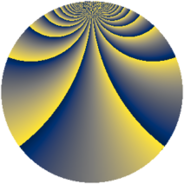# Properties

 Label 2240.2.bcLevel $2240$ Weight $2$ Character orbit 2240.bc Rep. character $\chi_{2240}(111,\cdot)$ Character field $\Q(\zeta_{4})$ Dimension $128$ Sturm bound $768$

# Related objects

## Defining parameters

 Level: $$N$$ $$=$$ $$2240 = 2^{6} \cdot 5 \cdot 7$$ Weight: $$k$$ $$=$$ $$2$$ Character orbit: $$[\chi]$$ $$=$$ 2240.bc (of order $$4$$ and degree $$2$$) Character conductor: $$\operatorname{cond}(\chi)$$ $$=$$ $$112$$ Character field: $$\Q(i)$$ Sturm bound: $$768$$

## Dimensions

The following table gives the dimensions of various subspaces of $$M_{2}(2240, [\chi])$$.

Total New Old
Modular forms 800 128 672
Cusp forms 736 128 608
Eisenstein series 64 0 64

## Trace form

 $$128q + O(q^{10})$$ $$128q - 8q^{11} + 16q^{23} + 16q^{29} + 16q^{37} + 8q^{43} - 80q^{51} + 16q^{53} - 40q^{67} + 64q^{71} - 128q^{81} + 32q^{91} + 40q^{99} + O(q^{100})$$

## Decomposition of $$S_{2}^{\mathrm{new}}(2240, [\chi])$$ into newform subspaces

The newforms in this space have not yet been added to the LMFDB.

## Decomposition of $$S_{2}^{\mathrm{old}}(2240, [\chi])$$ into lower level spaces

$$S_{2}^{\mathrm{old}}(2240, [\chi]) \cong$$ $$S_{2}^{\mathrm{new}}(112, [\chi])$$$$^{\oplus 6}$$$$\oplus$$$$S_{2}^{\mathrm{new}}(448, [\chi])$$$$^{\oplus 2}$$$$\oplus$$$$S_{2}^{\mathrm{new}}(560, [\chi])$$$$^{\oplus 3}$$